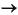ProgrammingConverting from Numeric to String

The functions listed in this table provide a number of ways to convert numeric data to character strings.

 Function Description Example `char` Convert a positive integer to an equivalent character. (Truncates any fractional parts.) `[72 105]``'Hi'` `int2str` Convert a positive or negative integer to a character type. (Rounds any fractional parts.) `[72 105]``'72 105'` `num2str` Convert a numeric type to a character type of the specified precision and format. `[72 105]``'72/105/'`(format set to `%1d/`) `mat2str` Convert a numeric type to a character type of the specified precision, returning a string MATLAB can evaluate. `[72 105]``'[72 105]'` `dec2hex` Convert a positive integer to a character type of hexadecimal base. `[72 105]``'48 69'` `dec2bin` Convert a positive integer to a character type of binary base. `[72 105]``'1001000 ` ` 1101001'` `dec2base` Convert a positive integer to a character type of any base from 2 through 36. `[72 105]``'110 151'`(base set to `8`)

Converting to a Character Equivalent

The `char` function converts integers to Unicode character codes and returns a string composed of the equivalent characters:

• ```x = [77 65 84 76 65 66];
char(x)
ans =
MATLAB
```

Converting to a String of Numbers

The `int2str`, `num2str`, and `mat2str` functions convert numeric values to strings where each character represents a separate digit of the input value. The `int2str` and `num2str` functions are often useful for labeling plots. For example, the following lines use `num2str` to prepare automated labels for the x-axis of a plot:

• ```function plotlabel(x, y)
plot(x, y)
str1 = num2str(min(x));
str2 = num2str(max(x));
out = ['Value of f from ' str1 ' to ' str2];
xlabel(out);
```

Converting to a Specific Radix

Another class of conversion functions changes numeric values into strings representing a decimal value in another base, such as binary or hexadecimal representation. This includes `dec2hex`, `dec2bin`, and `dec2base`.Searching and Replacing Converting from String to Numeric© 1994-2005 The MathWorks, Inc.